# RS Aggarwal Class 8 Solutions Chapter 10 Profit and Loss Ex 10A

## RS Aggarwal Class 8 Solutions Chapter 10 Profit and Loss Ex 10A

These Solutions are part of RS Aggarwal Solutions Class 8. Here we have given RS Aggarwal Solutions Class 8 Chapter 10 Profit and Loss Ex 10A.

Other Exercises

Question 1.
Solution:
(i) C.P. = Rs. 620
S.P. = Rs. 713
Gain = S.P. – C.P. = Rs. 713 – Rs. 620
= Rs. 93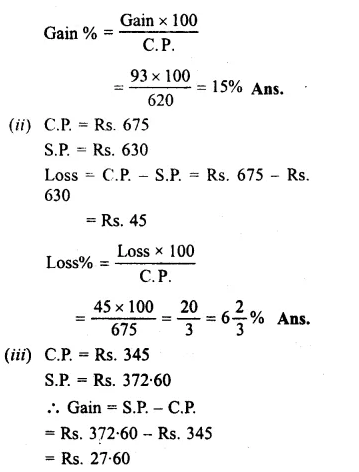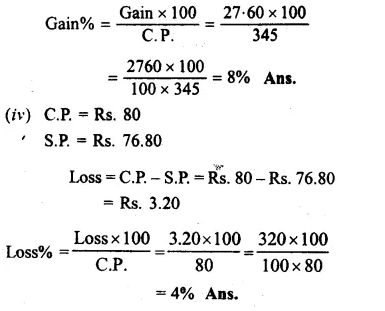Question 2.
Solution:
(i) C.P. = Rs. 1650
Gain% = 4%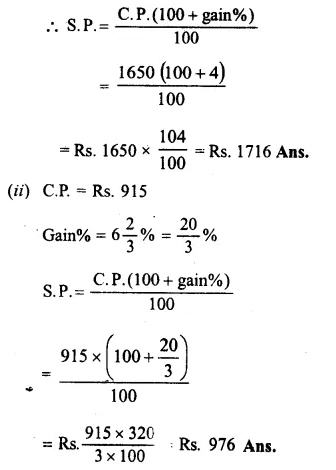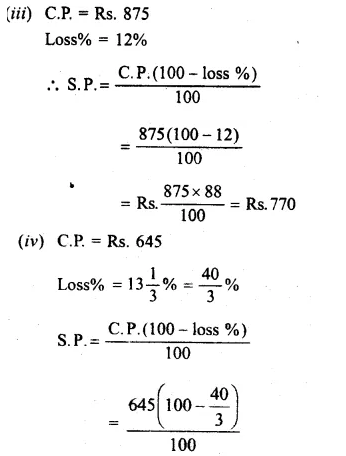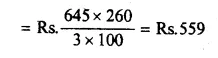Question 3.
Solution:
(i)S.P.= Rs 1596
Gain % = 12%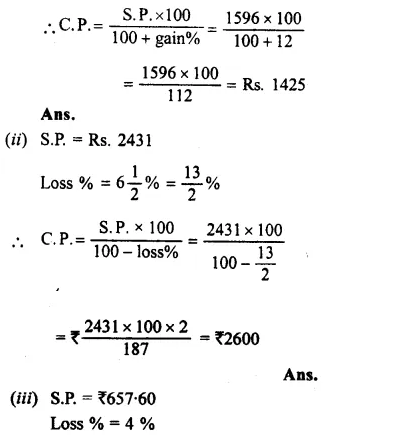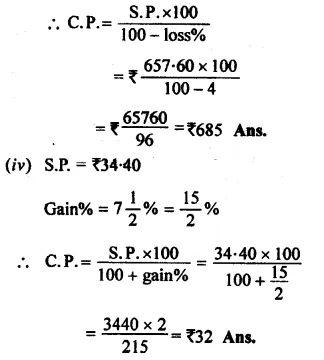Question 4.
Solution:
C.P. of iron safe = Rs 12160
Paid for its transportation = Rs 340
Total cost = Rs 12160 + Rs 340
= Rs 12500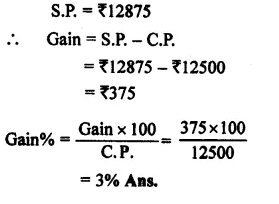Question 5.
Solution:
C.P. of car = Rs 73500
Overhead charges = Rs 10300 + 2600
= Rs 12900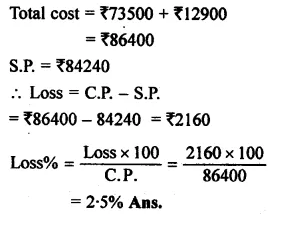Question 6.
Solution:
C.P. of 20 kg @ Rs 36 per kg.
= 20 x 36 = Rs 720
C.P. of 25 kg @ Rs32 per kg.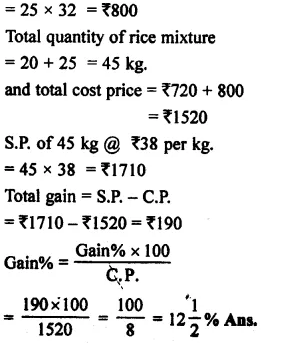Question 7.
Solution:
Ratio of the mixture = 5 : 2
Let 5 kg of coffee is mixed with 2 kg of chicory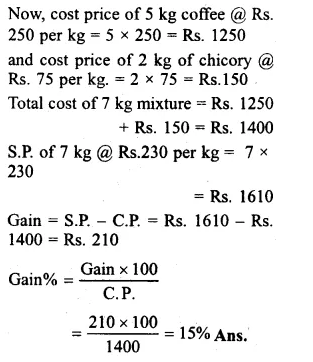Question 8.
Solution:
Let CR of 17 water bottles = Rs. 17
Then C.P. of one bottle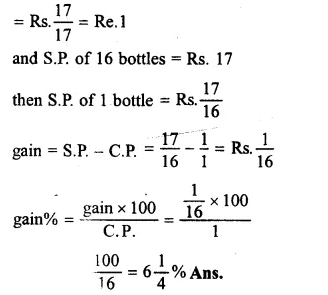Question 9.
Solution:
Let C.P. of 12 candIes =Rs. 12
C.P of 1 candle = Re. 1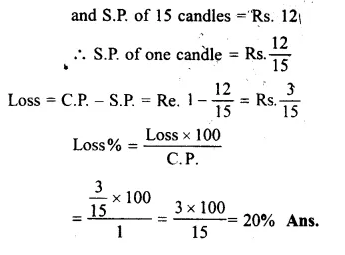Question 10.
Solution:
S.P. of 125 cassettes – gain = C.P. of 125 cassettes
=> S.P. of 125 cassettes – S.P. of 5 cassettes = C.P. of 125 cassettes
=> S.P. of 120 cassettes = C.P. of 125 cassettes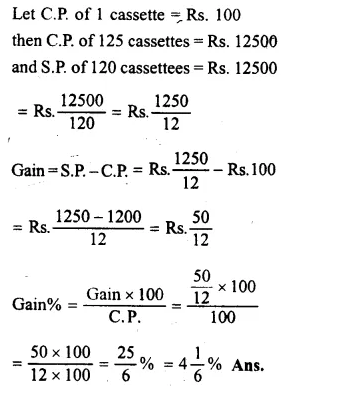Question 11.
Solution:
S.P. of 45 lemons = C.P. of 45 lemons – loss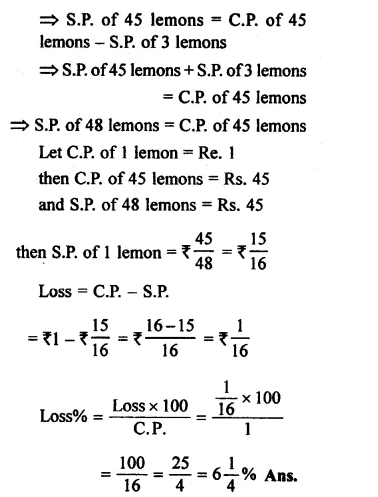Question 12.
Solution:
CP. of 6 oranges = Rs 20
and C.P of 1 orange = Rs $$\\ \frac { 20 }{ 6 }$$ = Rs $$\\ \frac { 10 }{ 3 }$$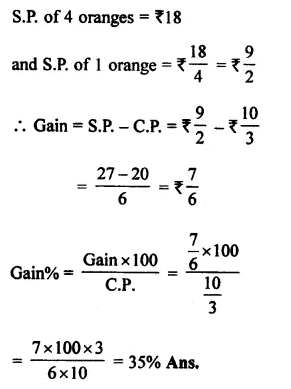Question 13.
Solution:
C.P. of 12 bananas = Rs 40
and C.P of 1 banana = Rs $$\\ \frac { 40 }{ 12 }$$ = Rs $$\\ \frac { 10 }{ 3 }$$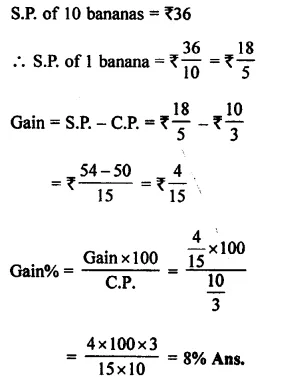Question 14.
Solution:
C.P. of 10 apples = Rs 75
and C.P of 1 apple = Rs $$\\ \frac { 75 }{ 10 }$$ = Rs $$\\ \frac { 15 }{ 2 }$$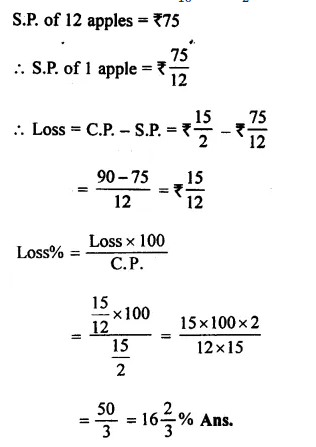Question 15.
Solution:
Let eggs purchased were = 3 x 16 = 48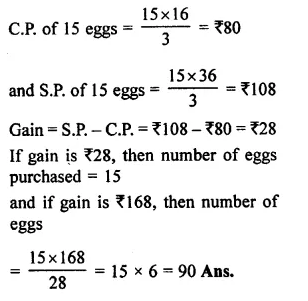Question 16.
Solution:
S.P. of camera = Rs 1080
C.P. of camera + gain = S.P. of camera
=> C.P. of camera + $$\\ \frac { 1 }{ 8 }$$ of C.P. = S.P. of camera
= $$\\ \frac { 9 }{ 8 }$$ x C.P. of camera = S.P. of camera = Rs 1080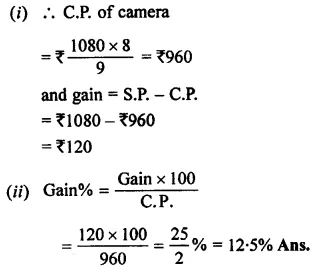Question 17.
Solution:
S.P. of a pen = Rs 54
Loss = $$\\ \frac { 1 }{ 10 }$$ of her outlay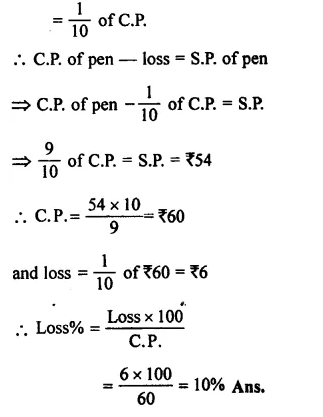Question 18.
Solution:
Let C.P. of table = Rs 100
In first case, loss = 10%
S.P. = Rs 100 – 10 = Rs 90
and in second case, gain = 10%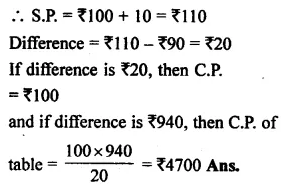Question 19.
Solution:
Let C.P. of chair = Rs 100
In first case, gain = 15%
then S.P. = Rs 100 + 15 = Rs 115
and in second case, gain = 8%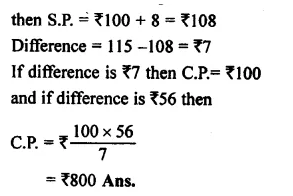Question 20.
Solution:
Let the C.P. of cycle = Rs 100
In first case, gain = 10%
then S.P. = Rs 100 + 10 = Rs 110
In second case, gain = 14%
Difference between their S.P.s = Rs 114 – Rs 110 = Rs 4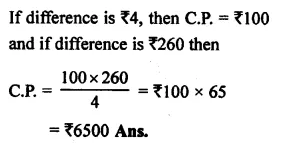Question 21.
Solution:
Cost price of 40 kg @ Rs 12.50 per kg.
= 40 x 12.50 = Rs 500
and cost price of 30 kg @ of Rs 14 per kg
= 30 x 14 = Rs 420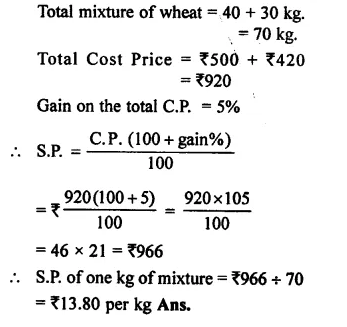Question 22.
Solution:
C.P. of first bat = Rs 840
Gain% = 15%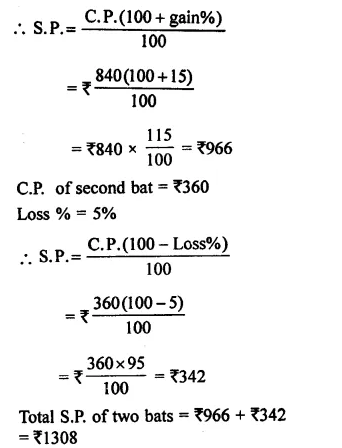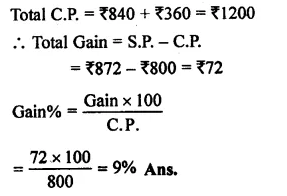Question 23.
Solution:
C.P. of first jean = Rs 1450
Gain% = 8%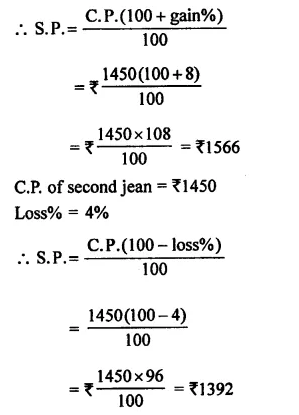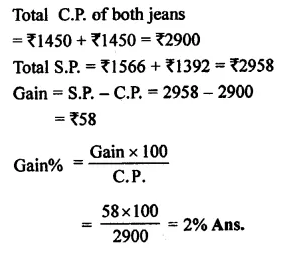Question 24.
Solution:
Total quantity of rice = 200 kg.
C.P. of 200 kg @ Rs 25 per kg.
= Rs 200 x 25 = Rs 5000
Gain% on total = 8%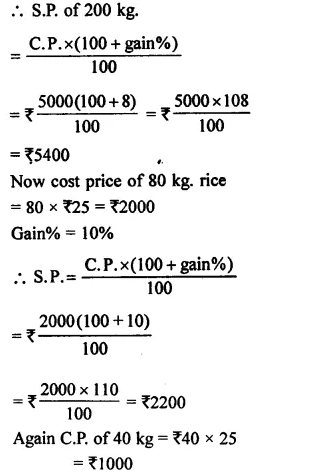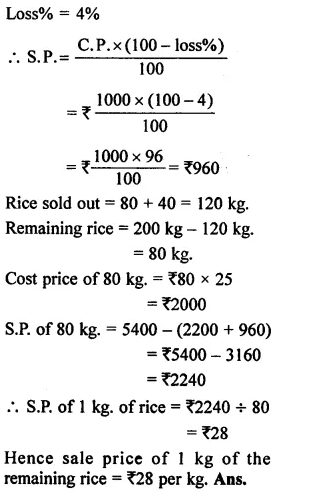Question 25.
Solution:
Let C.P. = Rs 100
then S.P. = $$\\ \frac { 6 }{ 5 }$$ of Rs 100Question 26.
Solution:
Let C.P. of flower vase = Rs 100
then S.P. $$\\ \frac { 5 }{ 6 }$$ of C.P. = $$\\ \frac { 5 }{ 6 }$$ of Rs 100
= Rs $$\\ \frac { 500 }{ 6 }$$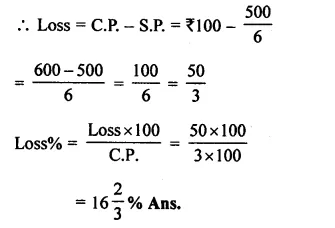Question 27.
Solution:
S.P. of a bouquet = Rs 322
Gain = 15%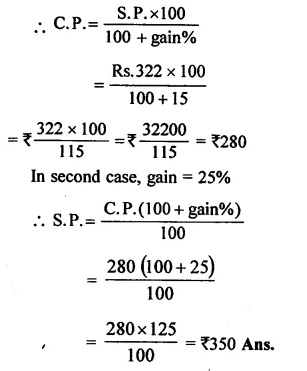Question 28.
Solution:
In first case,
S.P. of an umbrella = Rs 336
Loss% = 4%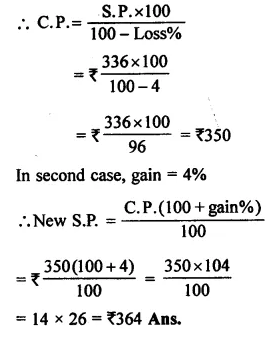Question 29.
Solution:
S.P. of Radio = Rs 3120
Loss% = 4%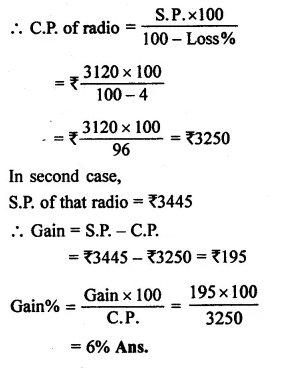Question 30.
Solution:
S.P. Of first sarees = Rs 1980
Loss% = 10%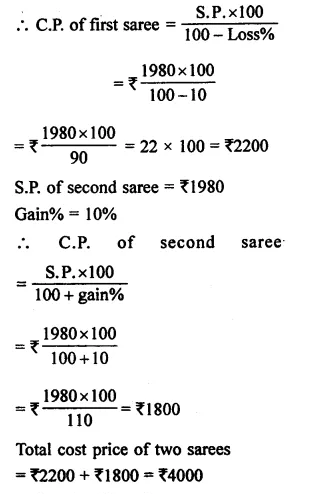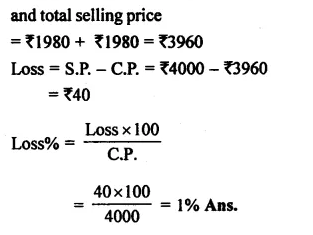Question 31.
Solution:
S.P. of first fan = Rs 1140
Gain% = 14%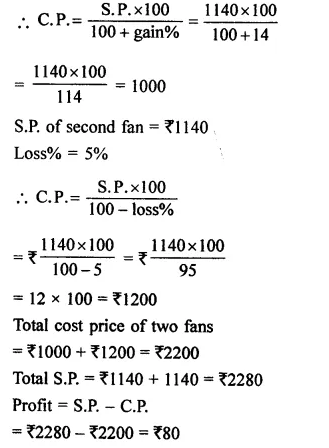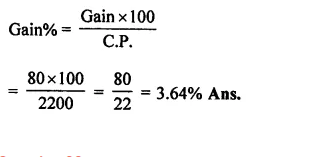Question 32.
Solution:
C.P. for Manoj = Rs 3990
or S.P. for Arun = Rs 3990
loss% = 5%
C.P for Arun
$$=\frac { S.P\times 100 }{ 100-losspercent} =\frac { 3990\times 100 }{ 100-5 }$$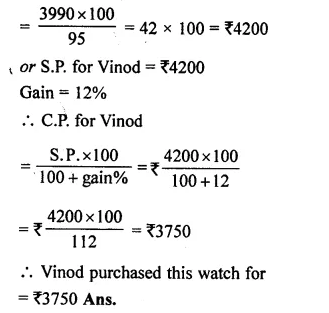Question 33.
Solution:
C.P. of plot of land = Rs 480000
C.P. of $$\\ \frac { 2 }{ 5 }$$th part = Rs 480000 x $$\\ \frac { 2 }{ 5 }$$
= Rs 192000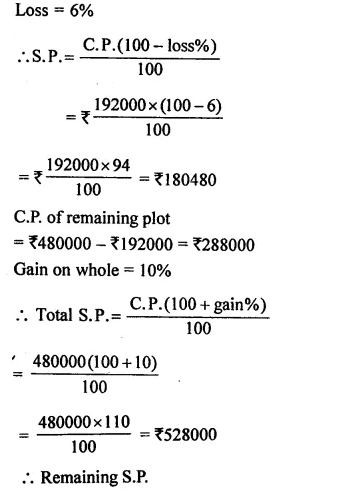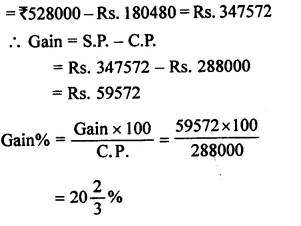Question 34.
Solution:
C.P. of sugar = Rs 4500
C.P. of $$\\ \frac { 1 }{ 3 }$$ of sugar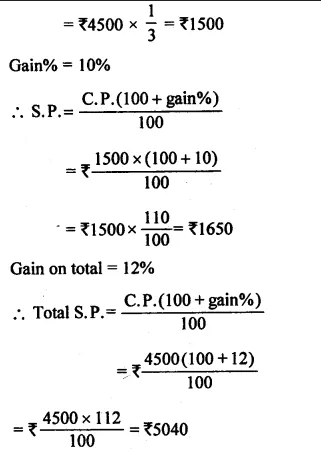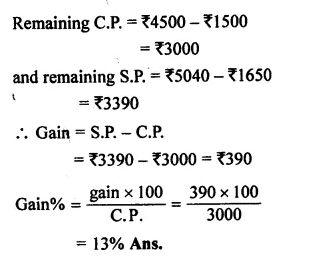Hope given RS Aggarwal Solutions Class 8 Chapter 10 Profit and Loss Ex 10A are helpful to complete your math homework.

If you have any doubts, please comment below. Learn Insta try to provide online math tutoring for you.